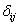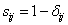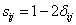### Introduction

Suppose we have four stars objects as shown in the figure below. Which ones of them are similar? Which ones of them are different?You may say that star A is similar to star C. Star A, B and C has the same size, while star A, C and D has the same color. Size and color are examples of features that can be measure.

Similarity is quite difficult to measure. Similarityis quantity that reflects the strength of relationship between two objects or two features. This quantity is usually having range of either -1 to +1 or normalized into 0 to 1. If the similarity between feature i and feature j is denoted by Sij, we can measure this quantity in several ways depending on the scale of measurement (or data type) that we have.

Distance measures dissimilarity. Dissimilarity measure the discrepancy between the two objects based on severalfeatures. Dissimilarity may also be viewed as measure of disorder between two objects. These features can be represented as coordinate of the object in the features space. There are many types of distance and similarity. Each of them has its own characteristics.

### Definition of Distance

Distance is a quantitative variable in general will satisfy the following at least the first three conditions below:

• dij >= 0 distance is always positive or zero;
• dij = 0 distance is zero if and only if it measured to itself;
• dij = dji distance is symmetry;
• dij <= dik + djk distance satisfy trangular inequality;

Distance is also called metric if it satisfies all above four conditions. Thus, because of the triangular inequality (condition 4), not all distance are metric, but all metric are distance.

### Relationship between similarity and dissimilarity

Let normalized dissimilarity between object i and object j is denoted by. The relationship between dissimilarity and similarity is given byfor similarity bounded by 0 and 1. When similarity is one (i.e. exactly similar), the dissimilarity is zero and when the similarity is zero (i.e. very different), the dissimilarity is one.

If the value of similarity has range of -1 and +1, and the dissimilarity is measured with range of 0 and 1, thenWhen dissimilarity is one (i.e. very different), the similarity is minus one and when the dissimilarity is zero (i.e. very similar), the similarity is one.

In many cases, measuring dissimilarity (i.e. distance) is easier than measuring similarity. Once we can measure the dissimilarity, we may easily normalize it and convert it to similarity measure.

### References & Resources

• http://people.revoledu.com/kardi/tutorial/Similarity/WhatIsSimilarity.html